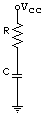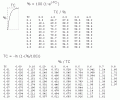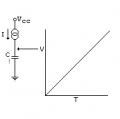# How to calculate timing for Constant Current capacitor charging?

#### Wendy

Joined Mar 24, 2008
23,320At some point we are introduced to Time Constants in our electronics education in charging a capacitor through a resistor. Which equals:

1TC=RxC

It is fundamental to all RC circuits. The 555 IC uses 1/3 Vcc to .67Vcc as its unit for timing, which works out to approx .69 TC. This is where the number .7 comes from in it timing formula.
From the formula shown we derive the classic charge curveI created this graph as a handy reference for myself. Since it was for myself I assumed the % meant % of Vcc.

Here is my question, Given a known charge rate in amps what is the %Vcc of the cap in a given time?

I do know the curve is linear similar to what is show below.Last edited:

#### AlbertHall

Joined Jun 4, 2014
12,293
Here is my question, Given a known charge rate in amps what is the %Vcc of the cap in a given time?
If you are charging with a constant current then VCC doesn't come into it. The voltage will rise at a constant rate (e.g. 1 volt per second).
V = IT/C

#### Wendy

Joined Mar 24, 2008
23,320
Yes, but what volts/Time units, I am trying to pin the math down

#### wayneh

Joined Sep 9, 2010
17,476
An amp is a coulomb per second. A farad is a volt per coulomb.

•Wendy

#### crutschow

Joined Mar 14, 2008
33,373
Yes, but what volts/Time units,
Δv/Δt = I/C (volts per second equals current divided by capacitance).

•Wendy

#### WBahn

Joined Mar 31, 2012
29,534
Here is my question, Given a known charge rate in amps what is the %Vcc of the cap in a given time?
Yes, but what volts/Time units, I am trying to pin the math down
The math comes directly from the defining relation for the capacitor.

Q = CV

I = dq/dt = C dv/dt

So

dv / dt = I/C

Note that C has units of farads, which are coulombs/volt. I has units of amperes, which are coulombs/sec. So dividing current by capacitance leaves you with units of volts/sec (or, in general, voltage/time).

If you want to relate this to a fraction of a particular current (Vcc or any other), then you just need to multiply this by 100%/Vcc and you get

d%/dt = (100%·I)/(C·Vcc)

#### wayneh

Joined Sep 9, 2010
17,476
A farad is a volt per coulomb.
Note that C has units of farads, which are coulombs/volt.
My bad, I should have looked it up because my off-the-top-of-my-head was backwards. My point was really that, if you follow your units, the math is simple.

#### Wendy

Joined Mar 24, 2008
23,320
Thank you everyone who stepped up to help me! When I was a young teen I studied electronics on my own to the point my college courses helped fill in the gaps. If the internet had existed then I would have probably gotten an EE Degree, It really helps when people are willing to explain concepts I missed first time around without being obnoxious about it, I have bookmarked this thread on my personal index for future reference. I suspect it will be as helpful for other people as it has for me. Thank You again. I try to pay it forward with my schematics and articles I write.

Last edited:

#### joeyd999

Joined Jun 6, 2011
5,037
For some reason, it has always been easiest for me to remember:

It=CV

Rearrange as necessary.

This only works for constant I.

•Bernard, atferrari and Wendy

#### Wendy

Joined Mar 24, 2008
23,320
I am in the middle of an article talking about Constant current source Beginners would not believe how many applications this linear function has for instrumentation circuits including DVM Voltage to digital conversions, I have to wonder what a CC on Inductor curve looks like?

#### OBW0549

Joined Mar 2, 2015
3,566
I have to wonder what a CC on Inductor curve looks like?
A constant current through an inductor results in zero voltage across it.

For a capacitor,

I = C * dV/dT

and for an inductor,

V = L * dI/dT.

#### Wendy

Joined Mar 24, 2008
23,320
But doing a little research it is still a linear line(curve) (1 volt change/sec) / coulomb until it is zero volts?

Another instrumentation application, Unless I am mistaken.

#### joeyd999

Joined Jun 6, 2011
5,037
I am in the middle of an article talking about Constant current source Beginners would not believe how many applications this linear function has for instrumentation circuits including DVM Voltage to digital conversions, I have to wonder what a CC on Inductor curve looks like?
In fact, I "discovered" It=CV in my youth when debugging an ICL7106 circuit. The problem -- which none of the actual engineers or technicians could figure out -- was that the integration cap was saturating at near full-scale input depending on the tolerance of the cap.

•Kjeldgaard and Wendy

#### WBahn

Joined Mar 31, 2012
29,534
But doing a little research it is still a linear line(curve) (1 volt change/sec) / coulomb until it is zero volts?

Another instrumentation application, Unless I am mistaken.
WHAT is still a linear line? Are you talking about a capacitor or an inductor, now?

Maybe I'm missing something, but there's no earth-shattering mystery here.

Many instruments for many decades have been based on things such as integrating a current onto a capacitor or the voltage across an inductor. Or, going the other way, turning the change in a signal voltage into a current signal via a capacitor or turning the change in a current into a voltage signal via an inductor.

#### Wendy

Joined Mar 24, 2008
23,320
The time for the flux to build in an inductor is still a straight line only going from the max voltage to zero volts, Just thinking of uses as I go along. A long while back I was thinking How to measure an inductance. This could actually make it easy. Previous to this I did not have the math tools to do this. Some skull sweat will be required on my part. Given my ever present brain fog, mental exercise is good for me. I am a bit excited about learning something new. May seem blase for someone who already knew it.

Last edited:

#### AnalogKid

Joined Aug 1, 2013
10,800
Yes, but what volts/Time units, I am trying to pin the math down
You can start with either the integral or differential form of the capacitor equation, and for a constant current the equation becomes:

E x C = i x t

E / t = i / C

If you have a specific volts/sec you want to achieve, you have one equation with two unknowns; there are an infinite number of i and C combinations that will work. Usually other design constraints narrow the field very quickly: available (or desired) charging current range, capacitor values already in inventory, etc.

ak

•cmartinez

#### Wendy

Joined Mar 24, 2008
23,320
For some reason, it has always been easiest for me to remember:

It=CV

Rearrange as necessary.

This only works for constant I.
Icc(seconds) = C V

So my interpretation is:
Icc = Constant current (in amps)
V = ΔV (total voltage change)

Did I interpret that right?

#### Wendy

Joined Mar 24, 2008
23,320
@WBahn
I'm going out on a limb, is this accurate?

V/L = Icc (seconds)

where V = (ΔV) Total voltage change
Icc = Constant Current (in Amps)
L = Inductance (in Henrys)

???

#### WBahn

Joined Mar 31, 2012
29,534
Icc(seconds) = C V

So my interpretation is:
Icc = Constant current (in amps)
V = ΔV (total voltage change)

Did I interpret that right?
Yes.

The constitutive equation for a linear capacitor is

Q = CV

If the voltage, Vo, at a certain charge, Qo changes, we have

Q = (Qo + ΔQ) = C(Vo + ΔV)

This yields

ΔQ = C·ΔV

Since ΔQ = ∫i(t)·dt, The integral over a period ΔT of a constant current Io is

ΔQ = Io·ΔT = C·ΔV